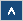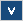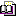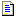Verilog Online HelpTable of ContentsBit-selectBlock StatementsBuilt-in PrimitivesCase StatementContinuous AssignmentsConversion FunctionsCommentsCompiler DirectivesConcatenationsConditional OperatorDelaysDisable StatementDisplay TasksEdge Sensitive PathExpression Bit LengthFile I/O FunctionsFunctionsIdentifiersIf StatementInteger ConstantsIntra-assignment Timing ControlsKeywordsLoop StatementsMemoriesmin:typ:max DelaysModule DeclarationModule InstantiationModule Path DeclarationModule Path PolarityNet Data TypesOperatorsParametersPart-selectPLA Modeling TasksProbabilistic Distribution FunctionsProcedural AssignmentsProcedural Continuous AssignmentsProcedural Timing ControlRange SpecificationReal ConstantsRegister Data TypesSimulation Control TasksSimulation Time FunctionsSpecify BlockState Dependent PathStochastic Analysis TasksStrengthsStringsStructured ProceduresTasksTimescale System TasksTiming Check TasksUDP DeclarationUDP InstantiationUDP State TableValue Change Dump (VCD) FileVectors

# UDP State Table

## Formal Definition

A UDP state table defines the behavior of UDP.

## Simplified Syntax

table

comb_input comb_input ... comb_input : output;

seq_input seq_input ... seq_input : current_state : next_state;

endtable

## Description

Each row of the state table defines the behavior of the UDP for a specific input combination. Each combination of inputs can be given only one time. It is illegal to define the same combination of inputs for different outputs.

The number of input values must match the number of UDP inputs in a port declaration.

The UDP state table for combinational and sequential UDPs is different. The combinational UDPs (Example 1) contain two fields: an input field and an output field. The sequential UDPs (Example 2) contain three fields: an input, a current state, and the next state (output) field.

The input field should contain a list of values (separated by white spaces), which should match the number of UDP input ports. The order of these ports is highly important. The first input port on the UDP port list matches the first input value in each row.

The rows of the state table can contain only 0, 1, unknown (x) value, and special characters. The high-impedance (z) value cannot be specified. The special characters are provided to increase readability of a description and to make it easier (Example 3).

 Symbol Interpretation Comments 0 Logic 0 1 Logic 1 x Unknown value Not permitted in output field. b Substitute for 0 and 1 Not permitted in output field. ? Substitute for 0, 1, and x Not permitted in output field. - No change Permitted only in output field of sequential UDPs. (vw) Transition from v to w value Permitted only in input field of sequential UDPs. v and w can be 0, 1, x, b, and ? * Same as (??) Any value change on input. Permitted only in input field of sequential UDPs. r Same as (01) Rising edge on input. Permitted only in input field of sequential UDPs. f Same as (10) Falling edge on input. Permitted only in input field of sequential UDPs. p Same as (01), (0x), (x1) Positive edge on input. Permitted only in input field of sequential UDPs. n Same as (10), (1x), (x0) Negative edge on input. Permitted only in input field of sequential UDPs.

Table 25: Summary of symbols

If some combinations of input signal values are not specified in a UDP state tables then the output value will be unknown (x).

Only one transition can appear in each row of sequential UDPs.

## Examples

Example 1

primitive mux (o, i3, i2, i1, i0, a1, a0);

output o;
input i3, i2, i1, i0, a1, a0;
table
// i3 i2 i1 i0 a1 a0 : o;
0 ? ? ? 1 1 : 0;
1 ? ? ? 1 1 : 1;
? 0 ? ? 1 0 : 0;
? 1 ? ? 1 0 : 1;
? ? 0 ? 0 1 : 0;
? ? 1 ? 0 1 : 1;
? ? ? 0 0 0 : 0;
? ? ? 1 0 0 : 1;
endtable
endprimitive

If any address bit (a1, a0) is unknown, then the output value will be unknown (because there is no row which describes the output value for this combination of address signals).

If a1 = 0 and a0 = 0 then the signal from input i0 is driven to output (value of others inputs does not matter).

If a1 = 0 and a0 = 1 then signal from input i1 is driven to the output.

If a1 = 1 and a0 = 0 then signal from input i2 is driven to the output.

If a1 = 1 and a0 = 1 then signal from input i3 is driven to the output.

Example 2

primitive special_d_ff (q, clk, m, d, rst, set);
output q;
reg q;
input clk, m, d, rst, set;
table
// clk m d rst set : current : next;
// positive edge on clk and normal mode (m = 0)
(01) 0 0 0 0 : ? : 0 ; // line 1
(0x) 0 0 0 0 : ? : 0 ; // line 2
(x1) 0 0 0 0 : ? : 0 ; // line 3
(01) 0 1 0 0 : ? : 1 ; // line 4
(0x) 0 1 0 0 : ? : 1 ; // line 5
(x1) 0 1 0 0 : ? : 1 ; // line 6
// positive edge on clk and negation mode (m = 1)
p 1 ? 0 0 : 0 : 1 ; // line 7
p 1 ? 0 0 : 1 : 0 ; // line 8
// negative edge on clk and any mode (m = 0 or m = 1)
n ? ? 0 0 : ? : - ; // line 9
// reset
? ? ? 1 ? : ? : 0 ; // line 10
// preset
? ? ? 0 1 : ? : 1 ; // line 11
// any changes on inputs with no changes on clk
? * ? ? ? : ? : - ; // line 12
? ? * ? ? : ? : - ; // line 13
? ? ? * ? : ? : - ; // line 14
? ? ? ? * : ? : - ; // line 15
endtable
endprimitive

This is 'd' flip-flop with an additional input, which can convert it into a modulo 2 counter.

If m = 0 then this UDP works as a normal 'd' flip-flop (lines 1-6), but if m = 1 then it negates its current value (lines 7 and 8). If any falling edge occurs on the clk input, then it will not change the output (line 9). If rst is 1, then the output will go to 0 (line 10). If the rst is 0 and set is 1, then the output will go to 1 (line 11 - it means that rst has higher priority than set).

Any changes on the data input will not cause any changes on output (lines 12-15).

Example 3

primitive circuit_1 (o, i1, i2, i3);
output o;
input i1, i2, i3;
table
0 0 0 : 1 ;
0 0 1 : 1 ;
0 0 x : 1 ;
0 1 0 : 1 ;
0 1 1 : 1 ;
0 1 x : 1 ;
1 0 0 : 0 ;
1 0 1 : 0 ;
1 0 x : 0 ;
1 1 0 : 1 ;
1 1 1 : 1 ;
1 1 x : 1 ;
x 0 0 : 0 ;
x 0 1 : 0 ;
x 0 x : 0 ;
x 1 0 : 0 ;
x 1 1 : 0 ;
x 1 x : 0 ;
endtable
endprimitive

primitive circuit_2 (o, i1, i2, i3);
output o;
input i1, i2, i3;
table
0 ? ? : 1 ;
1 0 ? : 0 ;
1 1 ? : 1 ;
x b ? : 0 ;
endtable
endprimitive

'Circuit_1' and 'circit_2' are the same circuit, but the behavior of the 'circuit_1' was specified without special characters.

## Important Notes

• Each row of sequential UDPs can contain only one transition.

• Special characters can make the UDP state table easier to read and write.

• Any undefined combination of inputs will set the output to x value.